# Non Verbal Reasoning - Classification

### Exercise :: Classification - Section 1

In each problem, out of the five figures marked (1), (2), (3), (4) and (5), four are similar in a certain manner. However, one figure is not like the other four. Choose the figure which is different from the rest.

16.

Choose the figure which is different from the rest.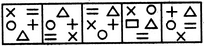(1)     (2)     (3)     (4)     (5)

 A. 1 B. 2 C. 3 D. 4 E. 5

Explanation:

Figure (4) has a rectangle in place of a '+' sign.

17.

Choose the figure which is different from the rest.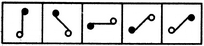(1)     (2)     (3)     (4)     (5)

 A. 1 B. 2 C. 3 D. 4 E. 5

Explanation:

All other figures can be rotated into each other.

18.

Choose the figure which is different from the rest.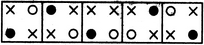(1)     (2)     (3)     (4)     (5)

 A. 1 B. 2 C. 3 D. 4 E. 5

Explanation:

In each one of the figures except fig. (3), the two crosses (x) appear in the diagonally opposite corners.

19.

Choose the figure which is different from the rest.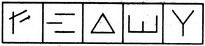(1)     (2)     (3)     (4)     (5)

 A. 1 B. 2 C. 3 D. 4 E. 5

Explanation:

Figure (4) is formed by four line segments while each one of the other figures is formed by three line segments.

20.

Choose the figure which is different from the rest.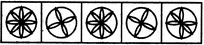(1)     (2)     (3)     (4)     (5)

 A. 1 B. 2 C. 3 D. 4 E. 5